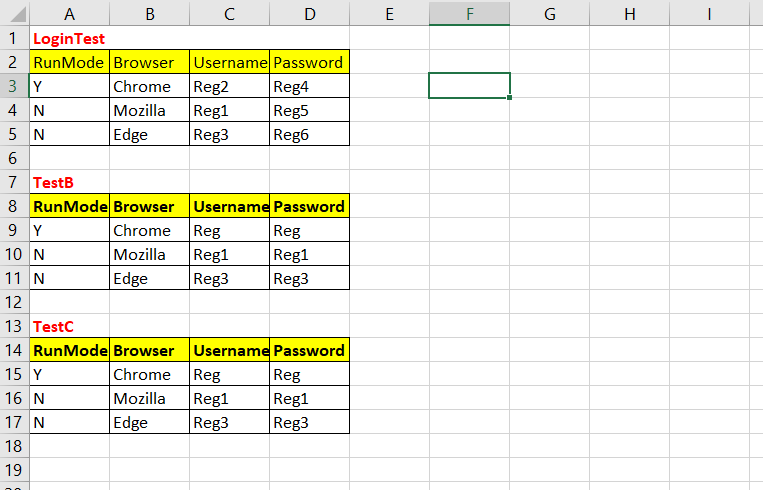Array index out of range is displayed reading the empty coloumn | Selenium Python Forum
A
Arvind C Posted on 21/08/2020

Temp file

def getdata():
list = []
xls = XLActions("C:\\Users\\Arvind\\Documents\\PythonKeyDrivenFramework\\Hybrid.xlsx")
#print(xls.get_CellData(2, 2,"Data"))

teststartrowindex = 0
#below loop is to check in each row if the testcase name matches
while not (xls.get_CellData(teststartrowindex, 0, "Data")) == testcasename:
teststartrowindex = teststartrowindex + 1

colrowindex = teststartrowindex + 1
datarowindex = teststartrowindex + 2

#below loop is to check in each row if the testcase section ends and there is empty row after each test case

#issue is observed here
datavalrows = 0
while not(xls.check_emptycolindex(datarowindex+datavalrows, 0, "Data")):
datavalrows = datavalrows + 1

#below loop is to check in each row if the testdata ends and there is empty coloumn
datavalcol = 0
while not(xls.check_emptycolindex(colrowindex,datavalcol,"Data")):
datavalcol = datavalcol + 1
dict = {}
for rownum in range(datarowindex,datarowindex+datavalrows):
for colnum in range(0,datavalcol):
xldatakeys = xls.get_CellData(colrowindex, colnum, "Data")
xldatavalue = xls.get_CellData(datarowindex,datavalcol,"Data")
dict[xldatakeys] = xldatavalue
list.append(dict)
return list
getdata()

Library File

import xlrd
import xlwt

class XLActions:

def __init__(self,path):
self.path = path
self.xlswriter = xlwt.Workbook(encoding="ascii")

def get_CellData(self,rowindex,colindex,sheetname):
return(sheet_name.cell_value(rowindex,colindex))

def check_emptycolindex(self,rowindex,colindex,sheetname):
s = cell.cell_type(rowindex,colindex)
if s == xlrd.XL_CELL_EMPTY:
return True
else:
return False

Above code is created after viewing video-3 in hybrid driven framework.

On executing above code an error "Array index out of range is displayed reading the empty coloumn".J
Jaspreet Replied on 22/08/2020

Hey,

You have to put the code in try-except block.

Like this :

``````maxRows = 0
try:
while not(xls.checkEmptyCell(constants.DATASHEET, dataStartRowIndex+maxRows, 0)):
maxRows = maxRows+1
except Exception:
pass``````

Make the changes like this in your code, and the error will be resolved. For further clarification you may watch the updated hybrid framework videos.

A
Arvind C Replied on 26/08/2020

Hello,

Thanks for the response the code is working now.

Regards,

Arvind

J
Jaspreet Replied on 27/08/2020

Hey,

Thank you for the update.